## Dividing Polynomials

### Learning Outcomes

• Divide polynomials by monomials
• Divide polynomials by binomials

The fourth arithmetic operation is division, the inverse of multiplication. Division of polynomials isn’t much different from division of numbers. In the exponential section, you were asked to simplify expressions such as: $\displaystyle\frac{{{a}^{2}}{{({{a}^{5}})}^{3}}}{8{{a}^{8}}}$. This expression is the division of two monomials. To simplify it, we divided the coefficients and then divided the variables. In this section we will add another layer to this idea by dividing polynomials by monomials, and by binomials.

## Divide a polynomial by a monomial

The distributive property states that you can distribute a factor that is being multiplied by a sum or difference.  Likewise, you can distribute a divisor that is being divided into a sum or difference. In this example, you can add all the terms in the numerator, then divide by $2$.

$\frac{\text{dividend}\rightarrow}{\text{divisor}\rightarrow}\,\,\,\,\,\, \frac{8+4+10}{2}=\frac{22}{2}=11$

Or you can first divide each term by $2$, then simplify the result.

$\frac{8}{2}+\frac{4}{2}+\frac{10}{2}=4+2+5=11$

Either way gives you the same result. The second way is helpful when you can’t combine like terms in the numerator.  Let’s try something similar with a binomial.

### Example

Divide. $\frac{9a^3+6a}{3a^2}$

In the next example, you will see that the same ideas apply when you are dividing a trinomial by a monomial. You can distribute the divisor to each term in the trinomial and simplify using the rules for exponents. As we have throughout the course, simplifying with exponents includes rewriting negative exponents as positive. Pay attention to the signs of the terms in the next example, we will divide by a negative monomial.

### Example

Divide. $\frac{27{{y}^{4}}+6{{y}^{2}}-18}{-6y}$

### Try It

Now, we ask you to think about what would happen if you were given a quotient like this to simplify: $\frac{27{{y}^{4}}+6{{y}^{2}}-18}{-6y+3}$.  You may be tempted to divide each term of ${27{{y}^{4}}+6{{y}^{2}}-18}$ individually by $-6y$, then $3$.  This would go against the order of operations because the division sign is a grouping symbol, and the addition in the denominator cannot be simplified anymore. The result is that no further operations can be performed with the tools we know.  We can, however, call into use a tool that you may have learned in gradeschool: long division.

## Polynomial Long Division

Recall how you can use long division to divide two whole numbers, say $900$ divided by $37$.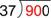First, you would think about how many $37$s are in $90$, as $9$ is too small. (Note: you could also think, how many $40$s are there in $90$.)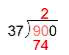There are two $37$s in $90$, so write $2$ above the last digit of  $90$. Two $37$s is $74$; write that product below the $90$.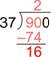Subtract: $90–74$ is $16$. (If the result is larger than the divisor, $37$, then you need to use a larger number for the quotient.)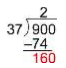Bring down the next digit $(0)$ and consider how many $37$s are in $160$.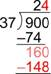There are four $37$s in $160$, so write the $4$ next to the two in the quotient. Four $37$s is $148$; write that product below the $160$.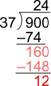Subtract: $160–148$ is $12$. This is less than $37$ so the $4$ is correct. Since there are no more digits in the dividend to bring down, you’re done.

The final answer is $24$ R$12$, or $24\frac{12}{37}$. You can check this by multiplying the quotient (without the remainder) by the divisor, and then adding in the remainder. The result should be the dividend:

$24\cdot37+12=888+12=900$

To divide polynomials, use the same process. This example shows how to do this when dividing by a binomial.

### Example

Divide: $\frac{\left(x^{2}–4x–12\right)}{\left(x+2\right)}$

Check this by multiplying:

$\left(x-6\right)\left(x+2\right)=x^{2}+2x-6x-12=x^{2}-4x-12$

### TRy It

Polynomial long division involves many steps.  Hopefully this video will help you determine what step to do next when you are using polynomial long division.

Let’s try another example. In this example, a term is “missing” from the dividend.

### Example

Divide: $\dfrac{\left(x^{3}–6x–10\right)}{\left(x–3\right)}$

The video that follows shows another example of dividing a third degree trinomial by a first degree binomial.   Note the “missing term” and pay close attention to how we work with it.

The process above works for dividing any polynomials no matter how many terms are in the divisor or the dividend and no matter what the degree of the polynomials are as long as the degree of the dividend is greater than or equal to the degree of the divisor. The main things to remember are:

• When subtracting, be sure to subtract the whole expression, not just the first term. This is very easy to forget, so be careful!
• Stop when the degree of the remainder is less than the degree of the dividend or when you have brought down all the terms in the dividend and your quotient extends to the right edge of the dividend.

The last video example shows how to divide a third degree trinomial by a second degree binomial.

## Summary

To divide a polynomial by a monomial, divide each term of the polynomial by the monomial. Be sure to watch the signs! Final answers should be written without any negative exponents.  Dividing polynomials by polynomials of more than one term can be done using a process very much like long division of whole numbers. You must be careful to subtract entire expressions, not just the first term. Stop when the degree of the remainder is less than the degree of the divisor. The remainder can be written using R notation, or as a fraction added to the quotient with the remainder in the numerator and the divisor in the denominator.

## Contribute!

Did you have an idea for improving this content? We’d love your input.